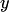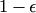# Conformal Prediction

Conformal prediction uses past experience to determine precise levels of confidence in new predictions. Given an error probability, together with a method that makes a point prediction of a label, it produces a set of labels, typically containing the point prediction, that also containswith probability. Conformal prediction can be applied to any method for producing point predictions: the nearest neighbours method, support vector machines, ridge regression, etc.

Conformal prediction is designed for the on-line setting, in which labels are predicted successively, each one being revealed before the next is predicted. The most novel and valuable feature of conformal prediction is that if the successive examples are sampled independently from the same distribution (randomness assumption), then the successive predictions will be rightof the time, even though they are based on an accumulating data set rather than on independent data sets.

The main classes of algorithms in conformal prediction and their variations are (the classes listed below are not disjoint):

Variations of conformal predictors adapted to probability forecasting include:

Conformal predictors, and related methods, can be used in environments that are more challenging than the on-line prediction protocol under the randomness assumption. This includes:

An interesting application of conformal prediction is to testing the randomness assumption (or a different on-line compression model).

There is some software implementing various methods of conformal prediction.

For predecessors of conformal prediction, see:

### Some open problems for conformal prediction:

Bibliography

• Vineeth N. Balasubramanian, Shen-Shyang Ho, and Vladimir Vovk, editors (2014). Conformal Prediction for Reliable Machine Learning: Theory, Adaptations, and Applications. Morgan Kaufmann, Chennai.
• Vladimir Vovk, Alexander Gammerman, and Glenn Shafer (2005). Algorithmic learning in a random world. Springer, New York.
• This question and answers to it on mathoverflow discuss the name "conformal prediction".